Anúncio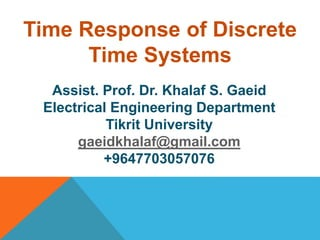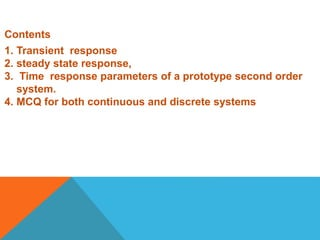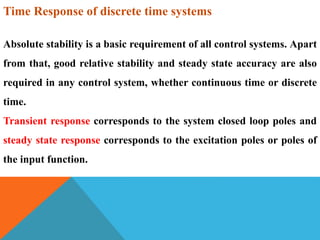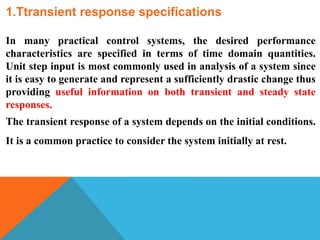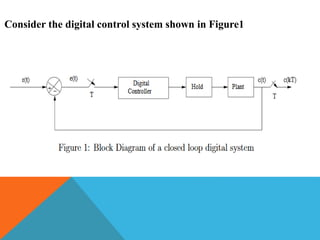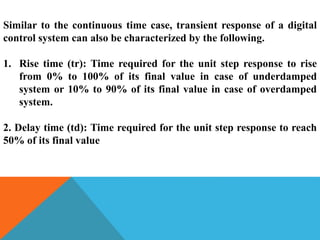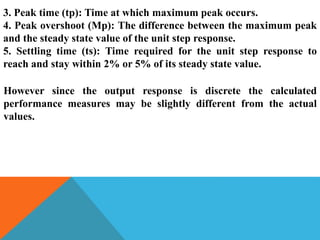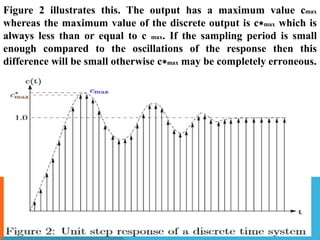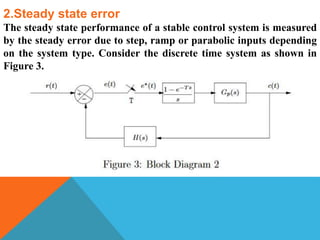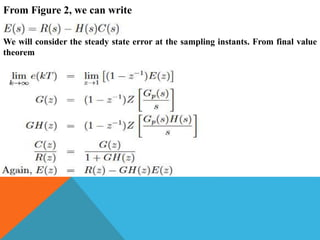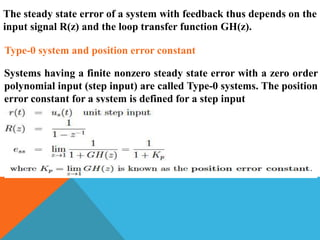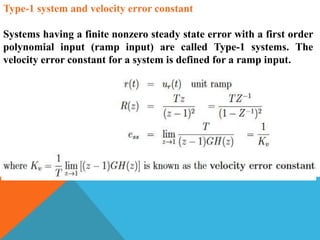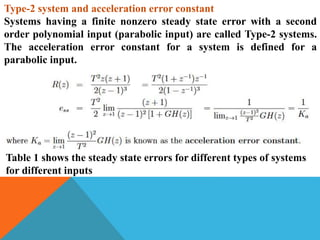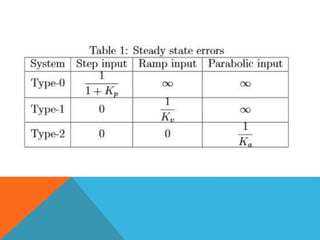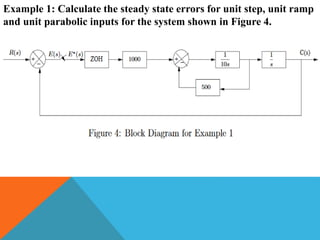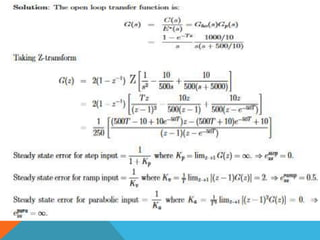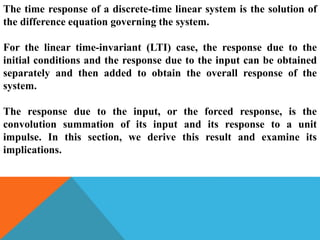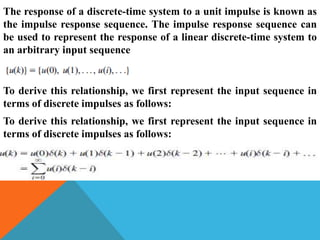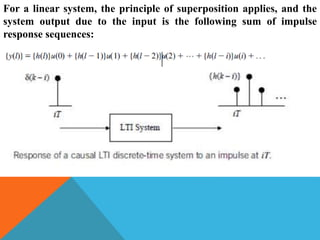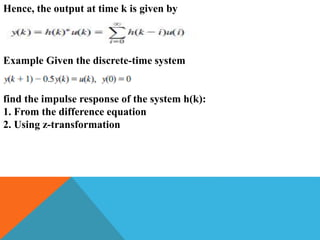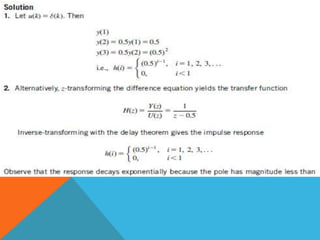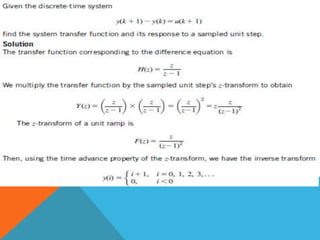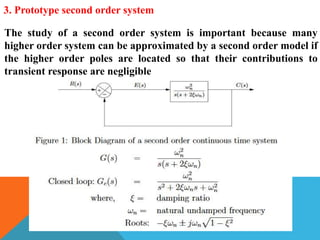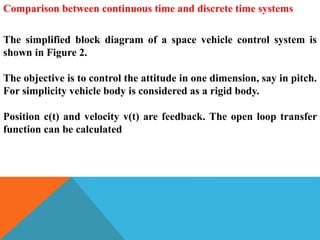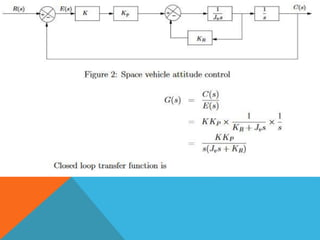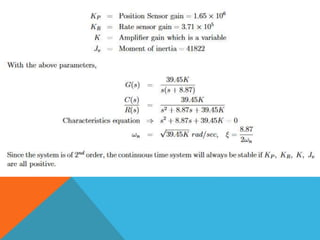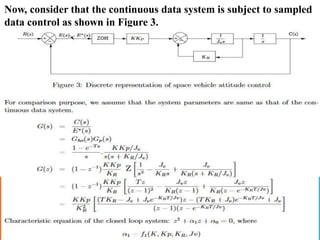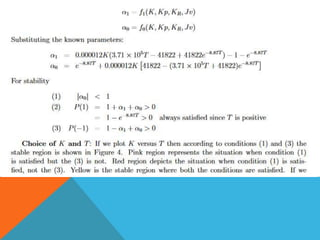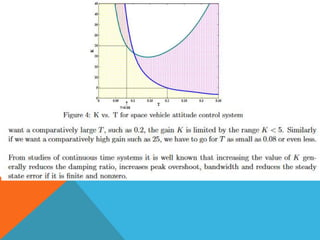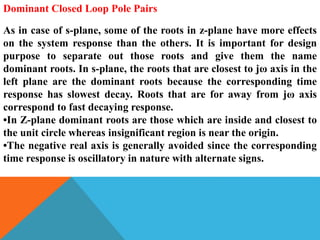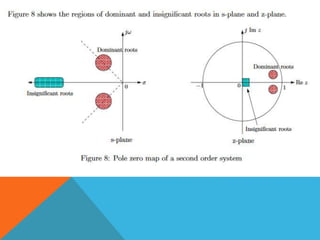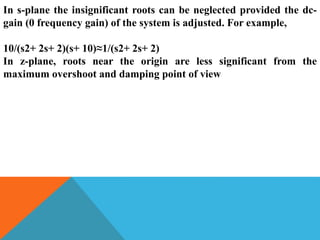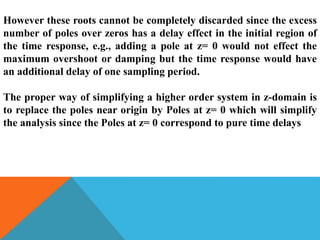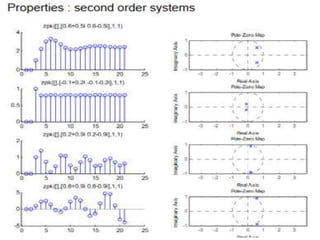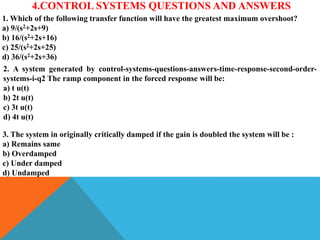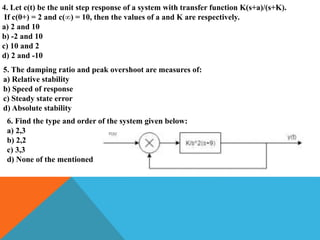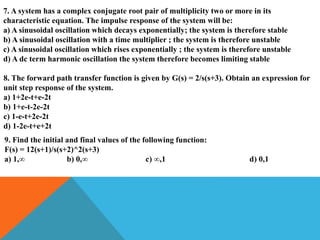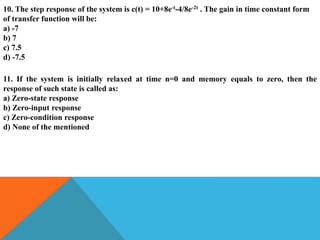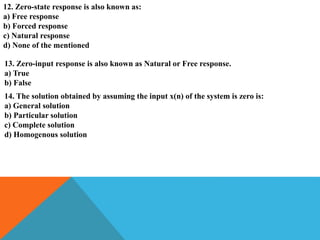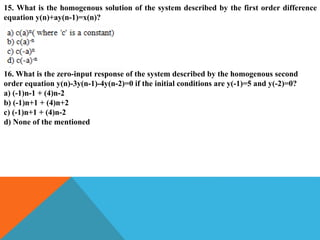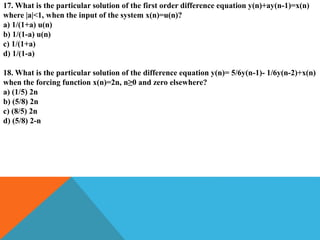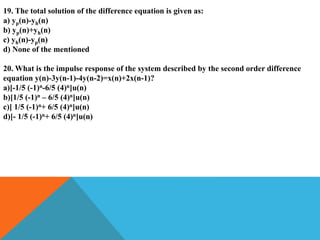1 de 43
Anúncio

### Time response of discrete systems 4th lecture

1. Assist. Prof. Dr. Khalaf S. Gaeid Electrical Engineering Department Tikrit University gaeidkhalaf@gmail.com +9647703057076 Time Response of Discrete Time Systems
2. 1. Transient response 2. steady state response, 3. Time response parameters of a prototype second order system. 4. MCQ for both continuous and discrete systems Contents
3. Time Response of discrete time systems Absolute stability is a basic requirement of all control systems. Apart from that, good relative stability and steady state accuracy are also required in any control system, whether continuous time or discrete time. Transient response corresponds to the system closed loop poles and steady state response corresponds to the excitation poles or poles of the input function.
4. 1.Ttransient response specifications In many practical control systems, the desired performance characteristics are specified in terms of time domain quantities. Unit step input is most commonly used in analysis of a system since it is easy to generate and represent a sufficiently drastic change thus providing useful information on both transient and steady state responses. The transient response of a system depends on the initial conditions. It is a common practice to consider the system initially at rest.
5. Consider the digital control system shown in Figure1
6. Similar to the continuous time case, transient response of a digital control system can also be characterized by the following. 1. Rise time (tr): Time required for the unit step response to rise from 0% to 100% of its final value in case of underdamped system or 10% to 90% of its final value in case of overdamped system. 2. Delay time (td): Time required for the unit step response to reach 50% of its final value
7. 3. Peak time (tp): Time at which maximum peak occurs. 4. Peak overshoot (Mp): The difference between the maximum peak and the steady state value of the unit step response. 5. Settling time (ts): Time required for the unit step response to reach and stay within 2% or 5% of its steady state value. However since the output response is discrete the calculated performance measures may be slightly different from the actual values.
8. Figure 2 illustrates this. The output has a maximum value cmax whereas the maximum value of the discrete output is c∗max which is always less than or equal to c max. If the sampling period is small enough compared to the oscillations of the response then this difference will be small otherwise c∗max may be completely erroneous.
9. 2.Steady state error The steady state performance of a stable control system is measured by the steady error due to step, ramp or parabolic inputs depending on the system type. Consider the discrete time system as shown in Figure 3.
10. From Figure 2, we can write We will consider the steady state error at the sampling instants. From final value theorem
11. The steady state error of a system with feedback thus depends on the input signal R(z) and the loop transfer function GH(z). Type-0 system and position error constant Systems having a finite nonzero steady state error with a zero order polynomial input (step input) are called Type-0 systems. The position error constant for a system is defined for a step input
12. Type-1 system and velocity error constant Systems having a finite nonzero steady state error with a first order polynomial input (ramp input) are called Type-1 systems. The velocity error constant for a system is defined for a ramp input.
13. Type-2 system and acceleration error constant Systems having a finite nonzero steady state error with a second order polynomial input (parabolic input) are called Type-2 systems. The acceleration error constant for a system is defined for a parabolic input. Table 1 shows the steady state errors for different types of systems for different inputs
14. Example 1: Calculate the steady state errors for unit step, unit ramp and unit parabolic inputs for the system shown in Figure 4.
15. The time response of a discrete-time linear system is the solution of the difference equation governing the system. For the linear time-invariant (LTI) case, the response due to the initial conditions and the response due to the input can be obtained separately and then added to obtain the overall response of the system. The response due to the input, or the forced response, is the convolution summation of its input and its response to a unit impulse. In this section, we derive this result and examine its implications.
16. The response of a discrete-time system to a unit impulse is known as the impulse response sequence. The impulse response sequence can be used to represent the response of a linear discrete-time system to an arbitrary input sequence To derive this relationship, we first represent the input sequence in terms of discrete impulses as follows: To derive this relationship, we first represent the input sequence in terms of discrete impulses as follows:
17. For a linear system, the principle of superposition applies, and the system output due to the input is the following sum of impulse response sequences:
18. Hence, the output at time k is given by Example Given the discrete-time system find the impulse response of the system h(k): 1. From the difference equation 2. Using z-transformation
19. 3. Prototype second order system The study of a second order system is important because many higher order system can be approximated by a second order model if the higher order poles are located so that their contributions to transient response are negligible
20. Comparison between continuous time and discrete time systems The simplified block diagram of a space vehicle control system is shown in Figure 2. The objective is to control the attitude in one dimension, say in pitch. For simplicity vehicle body is considered as a rigid body. Position c(t) and velocity v(t) are feedback. The open loop transfer function can be calculated
21. Now, consider that the continuous data system is subject to sampled data control as shown in Figure 3.
22. Dominant Closed Loop Pole Pairs As in case of s-plane, some of the roots in z-plane have more effects on the system response than the others. It is important for design purpose to separate out those roots and give them the name dominant roots. In s-plane, the roots that are closest to jω axis in the left plane are the dominant roots because the corresponding time response has slowest decay. Roots that are for away from jω axis correspond to fast decaying response. •In Z-plane dominant roots are those which are inside and closest to the unit circle whereas insignificant region is near the origin. •The negative real axis is generally avoided since the corresponding time response is oscillatory in nature with alternate signs.
23. In s-plane the insignificant roots can be neglected provided the dc- gain (0 frequency gain) of the system is adjusted. For example, 10/(s2+ 2s+ 2)(s+ 10)≈1/(s2+ 2s+ 2) In z-plane, roots near the origin are less significant from the maximum overshoot and damping point of view
24. However these roots cannot be completely discarded since the excess number of poles over zeros has a delay effect in the initial region of the time response, e.g., adding a pole at z= 0 would not effect the maximum overshoot or damping but the time response would have an additional delay of one sampling period. The proper way of simplifying a higher order system in z-domain is to replace the poles near origin by Poles at z= 0 which will simplify the analysis since the Poles at z= 0 correspond to pure time delays
25. 4.CONTROL SYSTEMS QUESTIONS AND ANSWERS 1. Which of the following transfer function will have the greatest maximum overshoot? a) 9/(s2+2s+9) b) 16/(s2+2s+16) c) 25/(s2+2s+25) d) 36/(s2+2s+36) 2. A system generated by control-systems-questions-answers-time-response-second-order- systems-i-q2 The ramp component in the forced response will be: a) t u(t) b) 2t u(t) c) 3t u(t) d) 4t u(t) 3. The system in originally critically damped if the gain is doubled the system will be : a) Remains same b) Overdamped c) Under damped d) Undamped
26. 4. Let c(t) be the unit step response of a system with transfer function K(s+a)/(s+K). If c(0+) = 2 and c(∞) = 10, then the values of a and K are respectively. a) 2 and 10 b) -2 and 10 c) 10 and 2 d) 2 and -10 5. The damping ratio and peak overshoot are measures of: a) Relative stability b) Speed of response c) Steady state error d) Absolute stability 6. Find the type and order of the system given below: a) 2,3 b) 2,2 c) 3,3 d) None of the mentioned
27. 7. A system has a complex conjugate root pair of multiplicity two or more in its characteristic equation. The impulse response of the system will be: a) A sinusoidal oscillation which decays exponentially; the system is therefore stable b) A sinusoidal oscillation with a time multiplier ; the system is therefore unstable c) A sinusoidal oscillation which rises exponentially ; the system is therefore unstable d) A dc term harmonic oscillation the system therefore becomes limiting stable 8. The forward path transfer function is given by G(s) = 2/s(s+3). Obtain an expression for unit step response of the system. a) 1+2e-t+e-2t b) 1+e-t-2e-2t c) 1-e-t+2e-2t d) 1-2e-t+e+2t 9. Find the initial and final values of the following function: F(s) = 12(s+1)/s(s+2)^2(s+3) a) 1,∞ b) 0,∞ c) ∞,1 d) 0,1
28. 10. The step response of the system is c(t) = 10+8e-t-4/8e-2t . The gain in time constant form of transfer function will be: a) -7 b) 7 c) 7.5 d) -7.5 11. If the system is initially relaxed at time n=0 and memory equals to zero, then the response of such state is called as: a) Zero-state response b) Zero-input response c) Zero-condition response d) None of the mentioned
29. 12. Zero-state response is also known as: a) Free response b) Forced response c) Natural response d) None of the mentioned 13. Zero-input response is also known as Natural or Free response. a) True b) False 14. The solution obtained by assuming the input x(n) of the system is zero is: a) General solution b) Particular solution c) Complete solution d) Homogenous solution
30. 15. What is the homogenous solution of the system described by the first order difference equation y(n)+ay(n-1)=x(n)? 16. What is the zero-input response of the system described by the homogenous second order equation y(n)-3y(n-1)-4y(n-2)=0 if the initial conditions are y(-1)=5 and y(-2)=0? a) (-1)n-1 + (4)n-2 b) (-1)n+1 + (4)n+2 c) (-1)n+1 + (4)n-2 d) None of the mentioned
31. 17. What is the particular solution of the first order difference equation y(n)+ay(n-1)=x(n) where |a|<1, when the input of the system x(n)=u(n)? a) 1/(1+a) u(n) b) 1/(1-a) u(n) c) 1/(1+a) d) 1/(1-a) 18. What is the particular solution of the difference equation y(n)= 5/6y(n-1)- 1/6y(n-2)+x(n) when the forcing function x(n)=2n, n≥0 and zero elsewhere? a) (1/5) 2n b) (5/8) 2n c) (8/5) 2n d) (5/8) 2-n
32. 19. The total solution of the difference equation is given as: a) yp(n)-yh(n) b) yp(n)+yh(n) c) yh(n)-yp(n) d) None of the mentioned 20. What is the impulse response of the system described by the second order difference equation y(n)-3y(n-1)-4y(n-2)=x(n)+2x(n-1)? a)[-1/5 (-1)n-6/5 (4)n]u(n) b)[1/5 (-1)n – 6/5 (4)n]u(n) c)[ 1/5 (-1)n+ 6/5 (4)n]u(n) d)[- 1/5 (-1)n+ 6/5 (4)n]u(n)